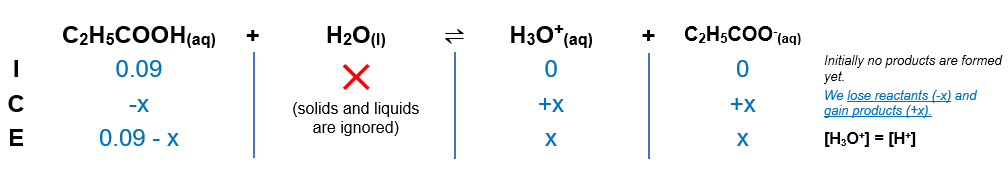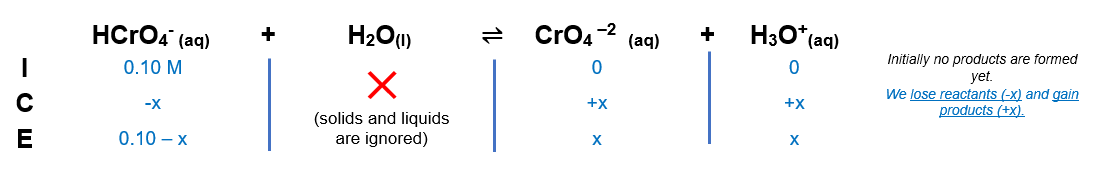# Problem: Calculate the pH of each of the following solutions (Ka and Kb values are given in Appendix D in the textbook).9.0×10−2 M propionic acid (C2H5COOH).0.100 M hydrogen chromate ion (HCrO−4).0.119 M pyridine (C5H5N).

###### FREE Expert Solution

We are asked to calculate the pH of each of the following solutions

A) 9.0×10−2 M propionic acid (C2H5COOH).

C2H5COOH is a weak acid and dissociates partially in aqueous solution.

C2H5COOH (aq) + H2O(l) H3O+(aq) + C2H5COO-(aq)                Ka = 9.0×10-2

Kb expression for C2H5COOH

The expression of Ka can be written like the equilibrium expression.

Solids and liquids are not included in equilibrium expressions.

ICE table for calculation of H3O+ ion concentration.

The concentrations can be determined using the ICE table.Plugging in the values (Ka gathered from online source; Ka = 1.3x10-5)

We need to find if we can neglect x in the denominator. We will take the ratio of [weak acid]/Ka to find this out.

$\frac{\left[\mathrm{HA}\right]}{\mathbf{Ka}}\mathbf{=}\frac{\mathbf{0}\mathbf{.}\mathbf{09}}{\mathbf{1}\mathbf{.}\mathbf{3}\mathbf{×}{\mathbf{10}}^{\mathbf{-}\mathbf{5}}}\mathbf{=}\mathbf{6923}$

Since this ratio is greater than 500, we can neglect x in the denominator.

x = [H3O+] = 1.082x10-3

pH of solution

pH of a solution is calculated by this formula.

Plugging in the H3O+ ion concentration to calculate pH

pH = 2.966

The pH of 9.0×10−2 M propionic acid (C2H5COOH) is 2.97

B) 0.100 M hydrogen chromate ion (HCrO−4).

Construct an ICE chart for the equilibrium reaction.

Since we’re dealing with a weak acid and Ka is an equilibrium expression, we will have to create an ICE chart to determine the equilibrium concentration of each species

We will use the first equilibrium reaction given:

HCrO4-  +  H2O (l) ⇋ CrO–2 (aq) + H3O(aq)                        Ka2 = 3.2 x 10–7Write the Ka is an equilibrium expression.

$\overline{){{\mathbf{K}}}_{{\mathbf{a}}}{\mathbf{=}}\frac{\mathbf{products}}{\mathbf{reactants}}}\phantom{\rule{0ex}{0ex}}{\mathbf{K}}_{\mathbf{a}}\mathbf{=}\frac{\left[{{\mathrm{CrO}}_{4}}^{2-}\right]\left[{H}_{3}{O}^{+}\right]}{\left[{{\mathrm{HCrO}}_{4}}^{-}\right]}$

Solids and liquids are not included in the expression

Calculate for the equilibrium concentration.

$\mathbf{3}\mathbf{.}\mathbf{2}\mathbf{×}{\mathbf{10}}^{\mathbf{-}\mathbf{7}}\mathbf{=}\frac{\left[\mathbf{x}\right]\left[\mathbf{x}\right]}{\left[\mathbf{0}\mathbf{.}\mathbf{100}\mathbf{-}\mathbf{x}\right]}\phantom{\rule{0ex}{0ex}}\mathbf{3}\mathbf{.}\mathbf{2}\mathbf{×}{\mathbf{10}}^{\mathbf{-}\mathbf{7}}\mathbf{=}\frac{{x}^{2}}{\left[0.100-x\right]}$

We need to find if we can neglect x in the denominator. We will take the ratio of [weak acid]/Ka to find this out.

$\frac{\left[{{\mathrm{HCrO}}_{4}}^{-}\right]}{\mathbf{Ka}}\mathbf{=}\frac{\mathbf{0}\mathbf{.}\mathbf{100}}{\mathbf{3}\mathbf{.}\mathbf{2}\mathbf{×}{\mathbf{10}}^{\mathbf{-}\mathbf{7}}}\mathbf{=}\mathbf{312500}$

Since this ratio is greater than 500, we can neglect x in the denominator.

x = [H3O+] = [HCrO4-] = 1.79x10-4

81% (361 ratings)###### Problem Details

Calculate the pH of each of the following solutions (Ka and Kb values are given in Appendix D in the textbook).

9.0×10−2 M propionic acid (C2H5COOH).

0.100 M hydrogen chromate ion (HCrO−4).

0.119 M pyridine (C5H5N).

Frequently Asked Questions

What scientific concept do you need to know in order to solve this problem?

Our tutors have indicated that to solve this problem you will need to apply the Weak Acids concept. If you need more Weak Acids practice, you can also practice Weak Acids practice problems.

What professor is this problem relevant for?

Based on our data, we think this problem is relevant for Professor Raymond's class at VCU.